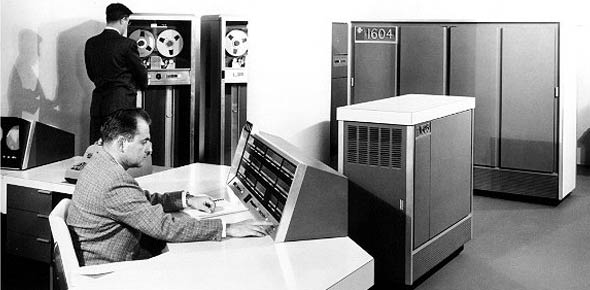# CDC 4b051 Practice Test

30 Questions | Total Attempts: 326SettingsCreate your own QuizEnd Of Course Practice Test

• 1.
(401) In ventilation work, what pressure is exerted outward against the interior surface of an enclosure?
• A.

Positive static

• B.

Negative static

• C.

Positive velocity

• D.

Negative velocity

• 2.
(401) After the fan of a ventilation systemis turned on, there is a conversion of
• A.

Total pressure of static pressure

• B.

Total pressure to velocity pressure

• C.

Velocity pressure to static pressure

• D.

Static pressure to velocity pressure

• 3.
(401) The value for velocity pressure in a ventilation system is
• A.

Always positive

• B.

Always negative

• C.

Negative on the blowing side

• D.

Negative on the exhausting side

• 4.
(402) Friction losses in a ventilation system can be reduced by increasing the
• A.

Hood size

• B.

Duct length

• C.

Duct velocity

• D.

Duct diameter

• 5.
(403)  A density factor of one means that the air you are dealing with is at
• A.

A high altitude

• B.

Standard conditions

• C.

Elevated temperature

• D.

High barometric pressure

• 6.
(403) which type of velocity refers to the ability of a ventilation system to remove contaminants from a work area?
• A.

Face velocity

• B.

Duct velocity

• C.

Capture velocity

• D.

Transport velocity

• 7.
(404) What are two parameters at a given point in ventilation systems that are needed to calculate the air flow volume?
• A.

Area and pressure

• B.

Area and velocity

• C.

Velocity and pressure

• D.

Diameter and velocity

• 8.
(404) Areas of ducts and hoods myst be expressed in
• A.

Inches

• B.

Linear feet

• C.

Square feet

• D.

Cubic feet per minute

• 9.
(405)  Which is the maximum amount of a certain chemical vapor that air can hold at a given temperature?
• A.

Vapor pressure

• B.

Explosive limit

• C.

Saturation concentration

• D.

Required air flow volumes

• 10.
(406) Other than good contaminant control, what is the biggest advantage of local exhaust over dilution ventilation?
• A.

Less noise

• B.

Lower operating costs

• C.

Better protection for equipment

• D.

• 11.
(407) Increasing the velocity of air through a slot hood without increasing the air flow volume most ofter results in
• A.

An increase in contaminant control

• B.

A decrease in contaminant control

• C.

An increase in system resistance

• D.

A decrease in system resiste

• 12.
(408) What may be indicated by a very strong flow coming in an open door of a room containing a local exhaust system?
• A.

• B.

Excessive crossdrafts

• C.

• D.

Proper discharge of airway wi

• 13.
(409) The range of acceptable values for the air flow volume on routine tests is within what persentage of the baseline?
• A.

25%

• B.

20%

• C.

15%

• D.

10%

• 14.
(410) At the beginning of a sound wave, the molecules near the source are drawn away from the surrounding air to form an area of lower atmospheric pressure.  This process is known as
• A.

Resonance

• B.

Compression

• C.

Rarefaction

• D.

Amplification

• 15.
(410) Which is a sound wave that can be characterized by a single frequency?
• A.

Pure Tone

• B.

White Noise

• C.

Complex sound

• D.

Reference sound

• 16.
(411) What is the characteristic of sound that we measure and report when we quantify sound?
• A.

Wave

• B.

Intensity

• C.

Weighting

• D.

Power level

• 17.
(412) What term would describe hearing loss due to an explosion?
• A.

Tinnitus

• B.

Acoustic Trauma

• C.

Threshold shift

• D.

Auditory Fatigue

• 18.
(413) Identifying equipment that produces hazardous moise at the operator's position is the purpose of what?
• A.

C/T calculation

• B.

Noise dosimetry

• C.

Sound level meter survey

• D.

Hearing conservation program

• 19.
(414) Assuming that altitude is not a factor, within what range should a sound level meter read when a 114 dB calibrator is placed over the microphone?
• A.

113.9-114.1

• B.

113.5-114.5

• C.

113-115

• D.

Exactly 114

• 20.
(414) If the sound level readings during one measurement difer by more that 6 dB(A), record the result as
• A.

The maximum level

• B.

The minimun level

• C.

3 dB(A) less than the maximum level

• D.

3 dB(A) more than the minimum level

• 21.
(415) What characteristic should a noise dosimeter have for measurements to be compared to Air Force standards?
• A.

Threshold of 85 dB(A)

• B.

Exchange rate of 3 dB

• C.

Criterion level of 90 dB(A)

• D.

Upper detection limit of a least 110 dB(A)

• 22.
(415) When would be considered the most appropriate time to conduct a noise dosimetry survey?
• A.

Just before or after a weekend

• B.

During a workplace inspection

• C.

If the workload is temporarily increased

• D.

When workdays are representative of normal shifts

• 23.
(416) How many meadurements do you take with an octave band analyzer during a survey?
• A.

5

• B.

7

• C.

10

• D.

12

• 24.
(417) How often are audiometric test booths monitored?
• A.

Monthly

• B.

Annually

• C.

Quarterly

• D.

Bi-annually

• 25.
(418) Noise dosimeters that indicate the daily dose as a percentage need to be converted to the
• A.

Compliance factor

• B.

Time weighted average

• C.

Average daily exposure

• D.

Daily equivalent sound level

Related TopicsBack to top
×

Wait!
Here's an interesting quiz for you.